Publicité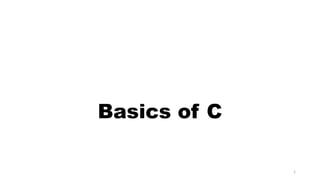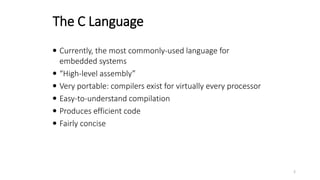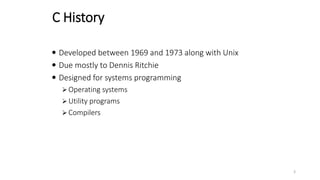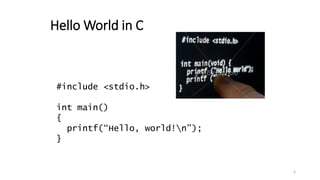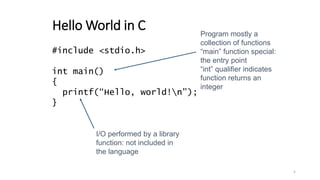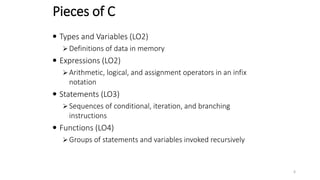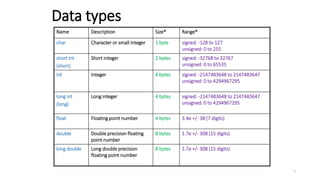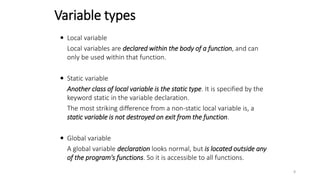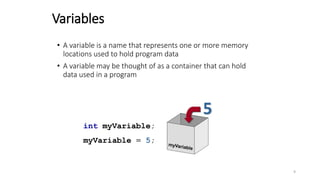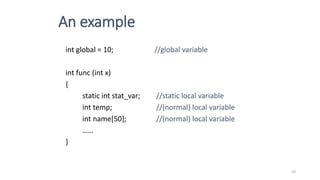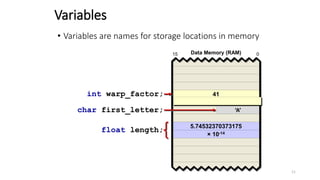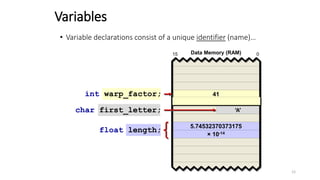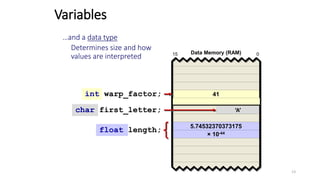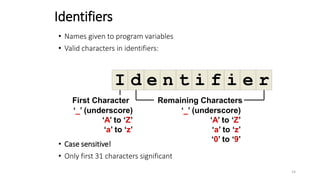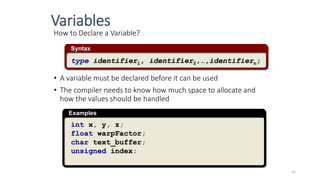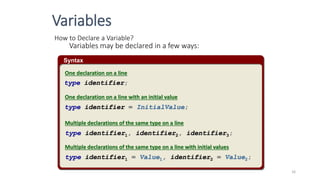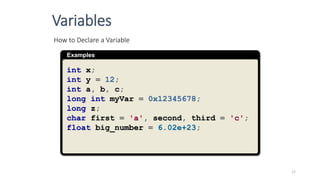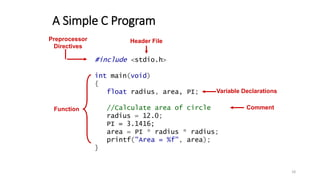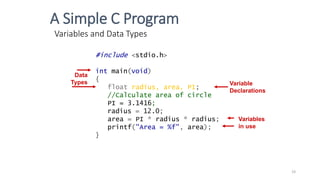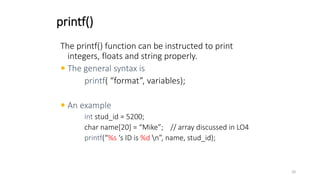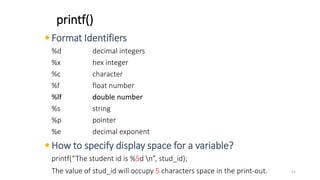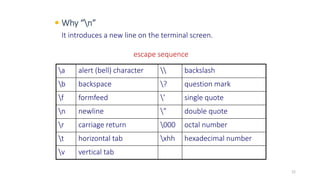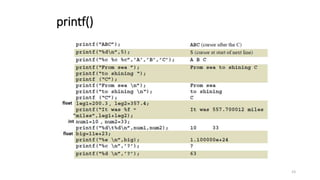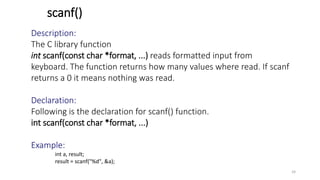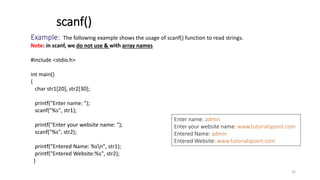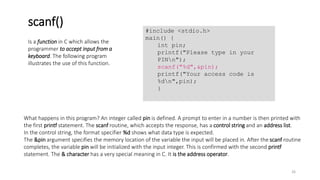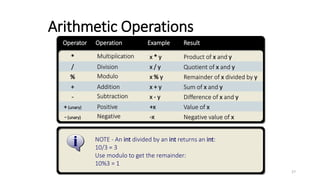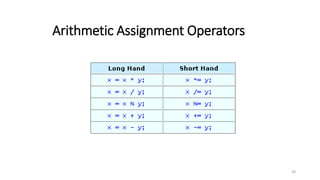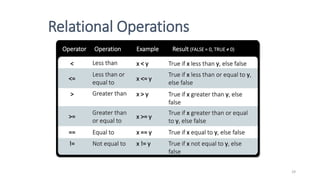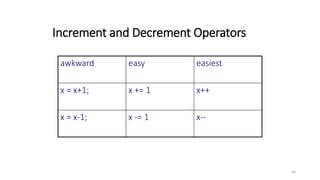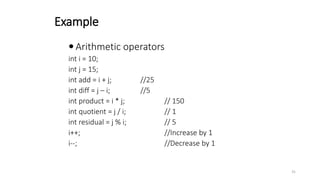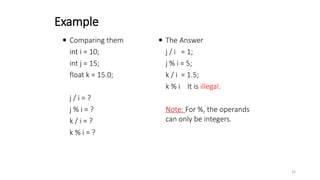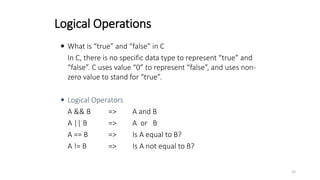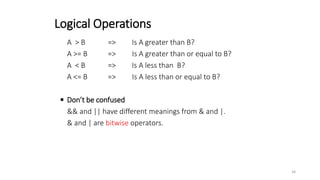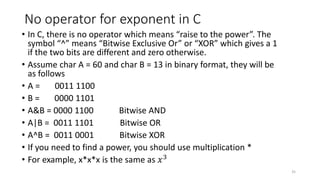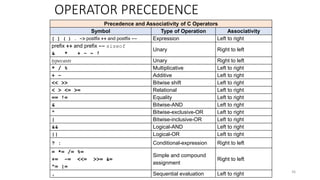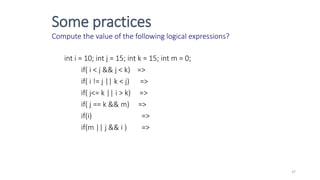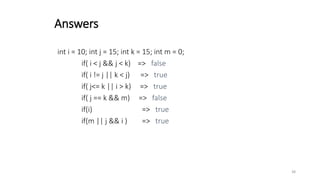1 sur 38
Publicité

### presentation_c_basics_1589366177_381682.pptx

1. Basics of C 1
2. The C Language  Currently, the most commonly-used language for embedded systems  “High-level assembly”  Very portable: compilers exist for virtually every processor  Easy-to-understand compilation  Produces efficient code  Fairly concise 2
3. C History  Developed between 1969 and 1973 along with Unix  Due mostly to Dennis Ritchie  Designed for systems programming  Operating systems  Utility programs  Compilers 3
4. Hello World in C #include <stdio.h> int main() { printf(“Hello, world!n”); } 4
5. Hello World in C #include <stdio.h> int main() { printf(“Hello, world!n”); } Program mostly a collection of functions “main” function special: the entry point “int” qualifier indicates function returns an integer I/O performed by a library function: not included in the language 5
6. Pieces of C  Types and Variables (LO2)  Definitions of data in memory  Expressions (LO2)  Arithmetic, logical, and assignment operators in an infix notation  Statements (LO3)  Sequences of conditional, iteration, and branching instructions  Functions (LO4)  Groups of statements and variables invoked recursively 6
7. Name Description Size* Range* char Character or small integer 1 byte signed: -128 to 127 unsigned: 0 to 255 short int (short) Short integer 2 bytes signed: -32768 to 32767 unsigned: 0 to 65535 int Integer 4 bytes signed: -2147483648 to 2147483647 unsigned: 0 to 4294967295 long int (long) Long integer 4 bytes signed: -2147483648 to 2147483647 unsigned: 0 to 4294967295 float Floating point number 4 bytes 3.4e +/- 38 (7 digits) double Double precision floating point number 8 bytes 1.7e +/- 308 (15 digits) long double Long double precision floating point number 8 bytes 1.7e +/- 308 (15 digits) Data types 7
8.  Local variable Local variables are declared within the body of a function, and can only be used within that function.  Static variable Another class of local variable is the static type. It is specified by the keyword static in the variable declaration. The most striking difference from a non-static local variable is, a static variable is not destroyed on exit from the function.  Global variable A global variable declaration looks normal, but is located outside any of the program's functions. So it is accessible to all functions. Variable types 8
9. Variables • A variable is a name that represents one or more memory locations used to hold program data • A variable may be thought of as a container that can hold data used in a program int myVariable; myVariable = 5; 5 9
10. An example int global = 10; //global variable int func (int x) { static int stat_var; //static local variable int temp; //(normal) local variable int name; //(normal) local variable …… } 10
11. 41 Variables 5.74532370373175 × 10-14 0 15 Data Memory (RAM) int warp_factor; float length; char first_letter; ‘A’ • Variables are names for storage locations in memory 11
12. char first_letter; int warp_factor; • Variable declarations consist of a unique identifier (name)… float length; 41 Variables 5.74532370373175 × 10-14 0 15 Data Memory (RAM) ‘A’ 12
13. char first_letter; float length; int warp_factor; 41 Variables 5.74532370373175 × 10-44 0 15 Data Memory (RAM) ‘A’ …and a data type Determines size and how values are interpreted 13
14. Identifiers • Names given to program variables • Valid characters in identifiers: • Case sensitive! • Only first 31 characters significant I d e n t i f i e r First Character ‘_’ (underscore) ‘A’ to ‘Z’ ‘a’ to ‘z’ Remaining Characters ‘_’ (underscore) ‘A’ to ‘Z’ ‘a’ to ‘z’ ‘0’ to ‘9’ 14
15. How to Declare a Variable? • A variable must be declared before it can be used • The compiler needs to know how much space to allocate and how the values should be handled Syntax type identifier1, identifier2,…,identifiern; Examples int x, y, z; float warpFactor; char text_buffer; unsigned index; Variables 15
16. Syntax How to Declare a Variable? Variables may be declared in a few ways: type identifier; type identifier = InitialValue; type identifier1, identifier2, identifier3; type identifier1 = Value1, identifier2 = Value2; One declaration on a line One declaration on a line with an initial value Multiple declarations of the same type on a line Multiple declarations of the same type on a line with initial values Variables 16
17. Examples How to Declare a Variable int x; int y = 12; int a, b, c; long int myVar = 0x12345678; long z; char first = 'a', second, third = 'c'; float big_number = 6.02e+23; Variables 17
18. A Simple C Program #include <stdio.h> int main(void) { float radius, area, PI; //Calculate area of circle radius = 12.0; PI = 3.1416; area = PI * radius * radius; printf("Area = %f", area); } Header File Function Variable Declarations Comment Preprocessor Directives 18
19. Variables and Data Types Variable Declarations Data Types Variables in use #include <stdio.h> int main(void) { float radius, area, PI; //Calculate area of circle PI = 3.1416; radius = 12.0; area = PI * radius * radius; printf("Area = %f", area); } A Simple C Program 19
20. printf() The printf() function can be instructed to print integers, floats and string properly.  The general syntax is printf( “format”, variables);  An example int stud_id = 5200; char name = “Mike”; // array discussed in LO4 printf(“%s ‘s ID is %d n”, name, stud_id); 20
21.  Format Identifiers %d decimal integers %x hex integer %c character %f float number %lf double number %s string %p pointer %e decimal exponent  How to specify display space for a variable? printf(“The student id is %5d n”, stud_id); The value of stud_id will occupy 5 characters space in the print-out. printf() 21
22.  Why “n” It introduces a new line on the terminal screen. a alert (bell) character backslash b backspace ? question mark f formfeed ’ single quote n newline ” double quote r carriage return 000 octal number t horizontal tab xhh hexadecimal number v vertical tab escape sequence 22
23. printf() 23
24. scanf() Description: The C library function int scanf(const char *format, ...) reads formatted input from keyboard. The function returns how many values where read. If scanf returns a 0 it means nothing was read. Declaration: Following is the declaration for scanf() function. int scanf(const char *format, ...) Example: int a, result; result = scanf("%d", &a); 24
25. Example: The following example shows the usage of scanf() function to read strings. Note: in scanf, we do not use & with array names #include <stdio.h> int main() { char str1, str2; printf("Enter name: "); scanf("%s", str1); printf("Enter your website name: "); scanf("%s", str2); printf("Entered Name: %sn", str1); printf("Entered Website:%s", str2); } scanf() Enter name: admin Enter your website name: www.tutorialspoint.com Entered Name: admin Entered Website: www.tutorialspoint.com 25
26. What happens in this program? An integer called pin is defined. A prompt to enter in a number is then printed with the first printf statement. The scanf routine, which accepts the response, has a control string and an address list. In the control string, the format specifier %d shows what data type is expected. The &pin argument specifies the memory location of the variable the input will be placed in. After the scanf routine completes, the variable pin will be initialized with the input integer. This is confirmed with the second printf statement. The & character has a very special meaning in C. It is the address operator. Is a function in C which allows the programmer to accept input from a keyboard. The following program illustrates the use of this function. scanf() #include <stdio.h> main() { int pin; printf("Please type in your PINn"); scanf("%d",&pin); printf("Your access code is %dn",pin); } 26
27. Negative -(unary) Subtraction - Positive +(unary) Modulo % Addition + NOTE - An int divided by an int returns an int: 10/3 = 3 Use modulo to get the remainder: 10%3 = 1 Multiplication * Division / Operator Result Operation Example -x x - y +x x % y x + y x * y x / y Negative value of x Difference of x and y Value of x Remainder of x divided by y Sum of x and y Product of x and y Quotient of x and y Arithmetic Operations 27
28. Arithmetic Assignment Operators 28
29. Operator Result (FALSE = 0, TRUE ≠ 0) Operation Example Equal to == Not equal to != Greater than > Greater than or equal to >= Less than < Less than or equal to <= x == y x != y x > y x >= y x < y x <= y True if x equal to y, else false True if x not equal to y, else false True if x greater than y, else false True if x greater than or equal to y, else false True if x less than y, else false True if x less than or equal to y, else false Relational Operations 29
30. Increment and Decrement Operators awkward easy easiest x = x+1; x += 1 x++ x = x-1; x -= 1 x-- 30
31. Example  Arithmetic operators int i = 10; int j = 15; int add = i + j; //25 int diff = j – i; //5 int product = i * j; // 150 int quotient = j / i; // 1 int residual = j % i; // 5 i++; //Increase by 1 i--; //Decrease by 1 31
32.  Comparing them int i = 10; int j = 15; float k = 15.0; j / i = ? j % i = ? k / i = ? k % i = ?  The Answer j / i = 1; j % i = 5; k / i = 1.5; k % i It is illegal. Note: For %, the operands can only be integers. Example 32
33. Logical Operations  What is “true” and “false” in C In C, there is no specific data type to represent “true” and “false”. C uses value “0” to represent “false”, and uses non- zero value to stand for “true”.  Logical Operators A && B => A and B A || B => A or B A == B => Is A equal to B? A != B => Is A not equal to B? 33
34. A > B => Is A greater than B? A >= B => Is A greater than or equal to B? A < B => Is A less than B? A <= B => Is A less than or equal to B?  Don’t be confused && and || have different meanings from & and |. & and | are bitwise operators. Logical Operations 34
35. No operator for exponent in C • In C, there is no operator which means “raise to the power”. The symbol “^” means “Bitwise Exclusive Or” or “XOR” which gives a 1 if the two bits are different and zero otherwise. • Assume char A = 60 and char B = 13 in binary format, they will be as follows • A = 0011 1100 • B = 0000 1101 • A&B = 0000 1100 Bitwise AND • A|B = 0011 1101 Bitwise OR • A^B = 0011 0001 Bitwise XOR • If you need to find a power, you should use multiplication * • For example, x*x*x is the same as 𝑥3 35
36. Precedence and Associativity of C Operators Symbol Type of Operation Associativity [ ] ( ) . –> postfix ++ and postfix –– Expression Left to right prefix ++ and prefix –– sizeof & * + – ~ ! Unary Right to left typecasts Unary Right to left * / % Multiplicative Left to right + – Additive Left to right << >> Bitwise shift Left to right < > <= >= Relational Left to right == != Equality Left to right & Bitwise-AND Left to right ^ Bitwise-exclusive-OR Left to right | Bitwise-inclusive-OR Left to right && Logical-AND Left to right || Logical-OR Left to right ? : Conditional-expression Right to left = *= /= %= += –= <<= >>= &= ^= |= Simple and compound assignment Right to left , Sequential evaluation Left to right OPERATOR PRECEDENCE 36
37. Some practices Compute the value of the following logical expressions? int i = 10; int j = 15; int k = 15; int m = 0; if( i < j && j < k) => if( i != j || k < j) => if( j<= k || i > k) => if( j == k && m) => if(i) => if(m || j && i ) => 37
38. int i = 10; int j = 15; int k = 15; int m = 0; if( i < j && j < k) => false if( i != j || k < j) => true if( j<= k || i > k) => true if( j == k && m) => false if(i) => true if(m || j && i ) => true Answers 38

1. 9
2. 11
3. 12
4. 13
5. 14
6. 15
7. 16
8. 17
9. 18
10. 19
11. 27
12. 29
Publicité